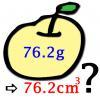#### You may also like### Far Horizon

An observer is on top of a lighthouse. How far from the foot of the lighthouse is the horizon that the observer can see?### Data Matching

Use your skill and judgement to match the sets of random data.### More or Less?

Are these estimates of physical quantities accurate?

# Constantly Changing

##### Age 14 to 16Challenge Level

The error $\Delta Z$ of the quantity $Z=\frac{A}{B}$ where $A$ and $B$ are independent satisfies $\left(\frac{\Delta Z}{Z}\right)^2 = \left(\frac{\Delta A}{A}\right)^2 + \left(\frac{\Delta B}{B}\right)^2$.

The error $\Delta Z$ of the quantity $Z=A+B$ where $A$ and $B$ are independent satisfies $(\Delta Z)^2 = (\Delta A)^2 + (\Delta B)^2$.

The error $\Delta Z$ of the quantity $Z=kA$  satisfies $(\Delta Z)^2 = (|k|\Delta A)^2$.

The error of $r' = \frac{m_p}{m_e} = 1836.15267...$ obeys:

\begin{align*}\left(\frac{\Delta r'}{r'}\right)^2 &= \left(\frac{8.3\times10^{-35}}{1.67...\times10^{-27}}\right)^2 + \left(\frac{4.5\times10^{-38}}{9.10...\times10^{-31}}\right)^2 \\&= 4.90\times^{-15}.\\\Rightarrow \Delta r' &= 1.29\times10^{-4}\end{align*}

Therefore, $r' = 1836.15267(13)$.

The proton/electron mass ratio, $r$, is $1836.152\, 672\, 4718(80)$. These values are consistent, as the given value for $r$ is within the error of $r'$. It appears the mass ratio is known to much greater accuracy than the individual masses.

The atomic mass of oxygen-16 is 15.99491461956(16)u, and the atomic mass of hydrogen-1 is 1.00782503207(10)u. The atomic mass of a water molecule is therefore $18.0104536837(26)u = 2.99072411(15)\times10^{-26}kg$. Therefore 1 mole of water weighs (mulitplying by Avogadro's constant) $1.80105647(13)\times10^{-2}kg$.

The energy in the mass of a mole of water is therefore $1.61870882(11)\times10^{15}J$.

Suppose a cup of tea has a volume of $200$ml and we need to raise its temperature from $20^{\circ}\mathrm{C}$ to $100^{\circ}\mathrm{C}$. Supposing there's no loss of energy in our heating system, the amount of energy in a mole of water could make $2.4\times10^{10}$ cups of tea!